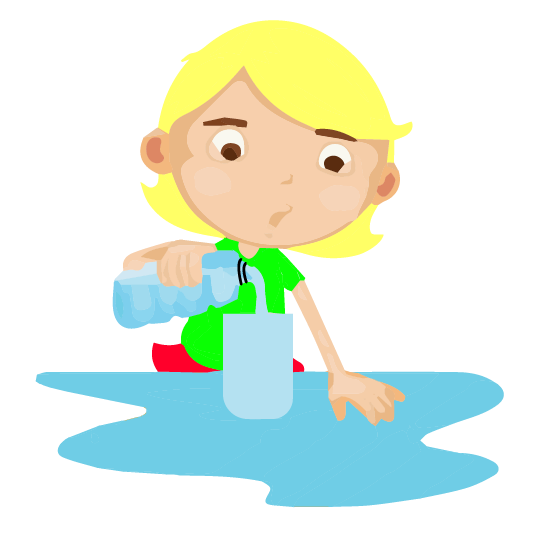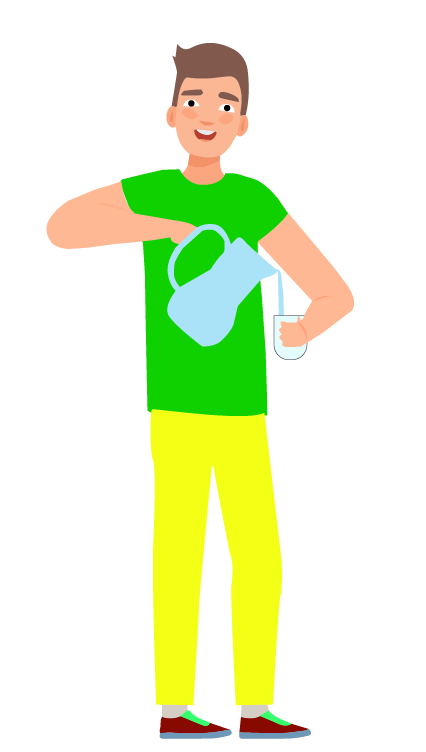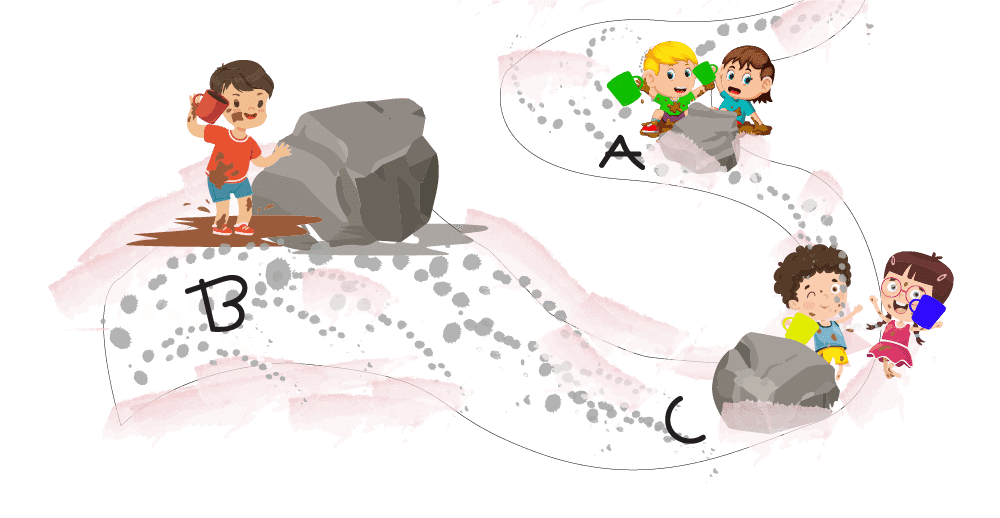Courses
Courses for Kids
Free study material
Offline Centres
More

# Jugs and Mugs Class 3 Notes CBSE Maths Chapter 11 (Free PDF Download)Last updated date: 04th Dec 2023
Total views: 165k
Views today: 3.65k

## Quick Preparation with CBSE Class 3 Maths Chapter 11 Jugs and Mugs Revision Notes

Chapter 11 Jugs and Mugs are the perfect illustration of volumes using simpler pictorial representations. Students will learn the less and more concept of volumes at this basic level. They will gain insights into the capacity of day-to-day objects and volumetric units.

By using the Jugs and Mugs revision notes prepared by the subject experts of Vedantu, you will gain more knowledge. Focus on the simplest explanation of concepts given by the experts covering every section. Learn how to understand and answer the questions in this chapter.

Also, check CBSE Class 3 Maths revision notes for other chapters:

## Revision Notes Class 3 Mathematics Chapter 11 - Jugs and Mugs

### What is Measurement?

• Measurement is a technique for determining an object's properties solely by comparing it to a standard quantity.

• Measurement is the most basic metric for expressing any quantity of objects, things, or events.

### The Measurement of Length

• Length is defined as the longest distance of anything which is measured from one point to another.

• The standard unit of length is the meter and in short, it is written as ‘m’.

• Other units such as centimetre, and millimetre are used as well and it all depends upon how large or small the distance or the length is.

• The relation between centimetre (cm) , metre (m) and kilometre (km) is

1 metre = 100 centimetre (cm) or 1 m = 100 cm

1 kilometre = 1000 metres or 1 km = 1000 m

### Converting Metre into Centimetre

• We know that 1 m = 100 cm.

• We have to just multiply the metres by 100 to convert metres into centimetres.

• Multiply metres by 100 and add the centimetres to convert metres and centimetres into centimetres.

• For example for converting 15 metres into centimetres we just have to multiply 15 by 100 hence 15 metres would become 1500 cm.

### Converting Centimetres into Metres

• We know that 1 m = 100 cm

• To convert a value from centimetres to metres we have to just divide the centimetres by 100. The quotient is the metres and the remainder gives the centimetres.

• For example, to convert 500 cm into m we have to divide 500 cm by 100 = 5.Here Quotient = 5 and remainder = 0.Hence 500 cm = 5m.

Solved question 1. Can a pen of length 450 mm fit inside a box of length 50 cm?

Ans: It is given that the length of the pencil =  450 mm and the length of the box = 50 cm. As both the units are different, we have to first convert 50 cm into mm

We know that,

1 metre = 100 centimetre (cm) or 1 m = 100 cm

1 cm = 10 mm

100 mm = 10 cm

50 cm = 50 x 10mm = 500 mm

As 450 mm is less than 500 mm we can say that the pen will fit in the box.

Solved question 2. A shopkeeper has 150 m of wire and he sold 25 metres of wire to customer A and 30 metres of wire to customer B. What is the length of wire left with him?

Ans: Total length of wire before selling = 150 m

Length of wire sold to customer A = 25 m

Length of wire sold to customer B = 30 m

The total length of wire left = Length of wire before selling - total length sold.

Total length of wire sold = 25 m + 30 m = 55 m

The total length of wire left =  150 - 55 = 95 m

Hence the length of wire left with the shopkeeper = 95 m

### Measurement of  Capacity

• Substances such as water, milk, kerosene, petrol etc are called liquids and the quantity or the amount of liquid a container can hold is called the capacity of the liquid.

•  Litres and millilitres are the units used to measure the capacity of the liquids.

• The short form of litres is L and millilitres is mL

• 1000 mL makes one litre or 1L = 1000 mL

• To convert Litres into millilitres we just have to multiply the number of litres present with 1000

• To convert mL into L we just have to divide the number of mL present with 1000

Solved question 1:  Whose jug holds more?

If Naima pours one glass of water into her jug, this is what it looks like.Naima pouring one glass of water

If Jeetu pours one glass of water into his jug, it looks like this.Jeetu pouring a glass of water

a. What are Naima and Jeetu doing?

Answer: They are filling their jugs with water.

b. Naima thinks she will have to pour around 3 glasses of water to fill the jug. What do you think?

Answer: She is right. She must pour three glasses of water to fill the jug.

c. Whose jug holds more water?

Answer: From the images, it is clear that Jeetu’s jug holds more water than Naima’s.

d. How many glasses of water do you think Jeetu should pour to fill his jug?

Answer: To fill his jug, Jeetu has to pour four glasses of water.

e. If Jeetu pours one more glass of water his jug will be around full.

Answer: If Jeetu pours one more glass of water, it won’t be around full, it would only be half-filled.

Solved question 2. Naseem and Abdul had to fill their pots each with water. Both pots were equally big and heavy so they went to the tap, again and again, filled their bottles and poured water into the pots. Naseem had to fill her bottle 16 times from the tap. But Abdul had to fill his bottle only 8 times.

a. Why did Naseem spend more time than Abdul?

Answer: Naseem had to go more times than Abdul as her bottle was smaller than Abdul's. Abdul had to go only 8 times as his bottle was bigger than Naseem's.

b. Naseem’s bottle can hold ____ (twice/half/three times) as much water as Abdul’s bottle.

Answer: Naseem's bottle is smaller than Abdul's and can hold half as much water as Abdul’s bottle.

Solved question 3. Pot B holds 11 glassfuls of water. Pot A holds twice as much water as pot B. How many glasses of water are needed to fill pot A?

Answer: It is given that the number of glasses of water pot B can hold is 11 glasses.

Pot A can hold twice as much water as pot B.

The total amount of water Pot A can hold = 11 x 2 = 22

Therefore we can say that pot A can hold 22 glasses of water.

Solved question 4. In a small town, the roads are full of potholes. Potholes are hollow in the road surfaces that are caused by over usage of the road.  Before it starts raining again, the children want to fill the holes with pebbles.

• The children bring pebbles in the mugs of the same size.

• With only 9 mugs of pebbles, Hole A gets filled.

• Hole B gets filled with 18 mugs of pebbles. Hole C gets filled with 12 mugs of pebbles.

View the image below and answer the following questions.Potholes filled with pebbles

a. Mark A, B and C on the right hole in the picture.Potholes filled with pebbles marked

b. Which are the biggest potholes among A, B and C?

Answer: From the image, it is clear that plot B is the biggest of all the three.

c. If jugs are used, hole A gets filled with 5 jugs. How many jugs of pebbles are needed to fill hole B?

Answer:  It is given that only 9 mugs of pebbles fill hole A whereas only 5 jugs are required to fill hole A.

The capacity of 9 mugs = The capacity of 5 jugs.

The number of mugs required to fill hole B = 9 x 2 = 18

So, therefore, the number of jugs required to fill pothole B = 2 x 5 = 10

Therefore, 10 jugs are required to fill pothole B.

### Practice Questions:

Q1.Aman drinks 9L 750 mL coffee and Raju drinks 7L 800 mL coffee in a week.  How much more coffee does Aman drink than Raju.

Q2.There are two containers of milk. Container A has a total of 10 L 250 mL milk and container B has 8L and 350 mL of milk. Find the total quantity of milk present in both containers.

Q3:A shopkeeper sells 12L 500 mL of olive oil on Monday, 3L 200 mL on Tuesday and 14L 200mL on Wednesday. Find the total amount of oil he sold in three days.

Ans 1. Aman drinks 1L 950 mL coffee more than Raju.

Hint: It is easy to solve this problem if one knows the concept of the capacity of liquids and also how to find the difference between the two capacities given.

Ans 2. Container A and container B have a total of 18L 600 mL of milk in them.

Hint: To solve this problem, students should know the concept of measurement of capacity and how to add two quantities when it's given.

Ans 3. The shopkeeper sold a total of 29L 900mL of olive oil in three days.

Hint:  To solve this problem, students should know the concept of measurement of capacity and how to add three quantities when it's given.

## Importance of CBSE Class 3 Maths Chapter 11 Jugs and Mugs

The composition of concepts in the form of pictures and illustrations makes this chapter quite important for the students in the following ways.

• An easy introduction to the concepts of volume using daily objects are provided.

• Explanations of concepts have been given in an easy way for quicker comprehension of the volume concepts.

• It initiates the formation of a foundation of units used for expressing volumes in NCERT.

• It also develops logical reasoning skills within Class 3 students.

• The questions in the sections and exercises are formulated in a puzzle format. Students will love to solve these puzzles to gain in-depth knowledge of volumes and conventional containers.

• They will also learn how to compare jugs, mugs, and filling pots in terms of volume in different situations.

• The problems included in the exercises provide a way for experiential learning for the students.

## Benefits of Vedantu’s Jugs and Mugs Worksheet and Revision Notes

• Concise elaboration of Jugs and Mugs summary for all sections

• Easy-to-comprehend language used for explaining concepts and problems

• Faster turnaround time for revising this chapter using the notes

• The easiest way to recall concepts, problem-solving methods, etc before an exam

• Sharpening problem-solving skills by using the Jugs and Mugs Class 3 worksheets compiled by the experts.

• Evaluation of logical reasoning and problem-solving skills based on the outcomes of solving water in water out problems.

• Quick referencing to the revision notes at your convenience makes study times more fruitful.

Get the free PDF versions of the revision notes of Class 3 Chapter 11 Maths along with the worksheets. Revise the chapter by referring to the easy explanation of concepts done by the experts. Understand how to match the right pairs and other problems using the worksheets and their answers. Develop your concepts and score well in the exams.

## FAQs on Jugs and Mugs Class 3 Notes CBSE Maths Chapter 11 (Free PDF Download)

1. How can you find out whose jug holds more water?

Compare both the cases after reading the information given in the description. You will understand whose jug will hold more water. Go through the problem, read the lines, and check how the experts have explained them in the NCERT Solutions for Class 3 Maths Chapter 11.

2. What is the objective of Class 3 Maths Chapter 11?

The prime objective of this chapter is to develop the idea of volumes, and containers, and to compare volumes of different objects among the students. They will learn how to compare different containers and their volumes along with units.

3. How can I prepare this chapter using the revision notes?

Focus on the chapter and solve all the questions given. Proceed to solve the worksheets on your own and then check the revision notes for a quick recollection of concepts.